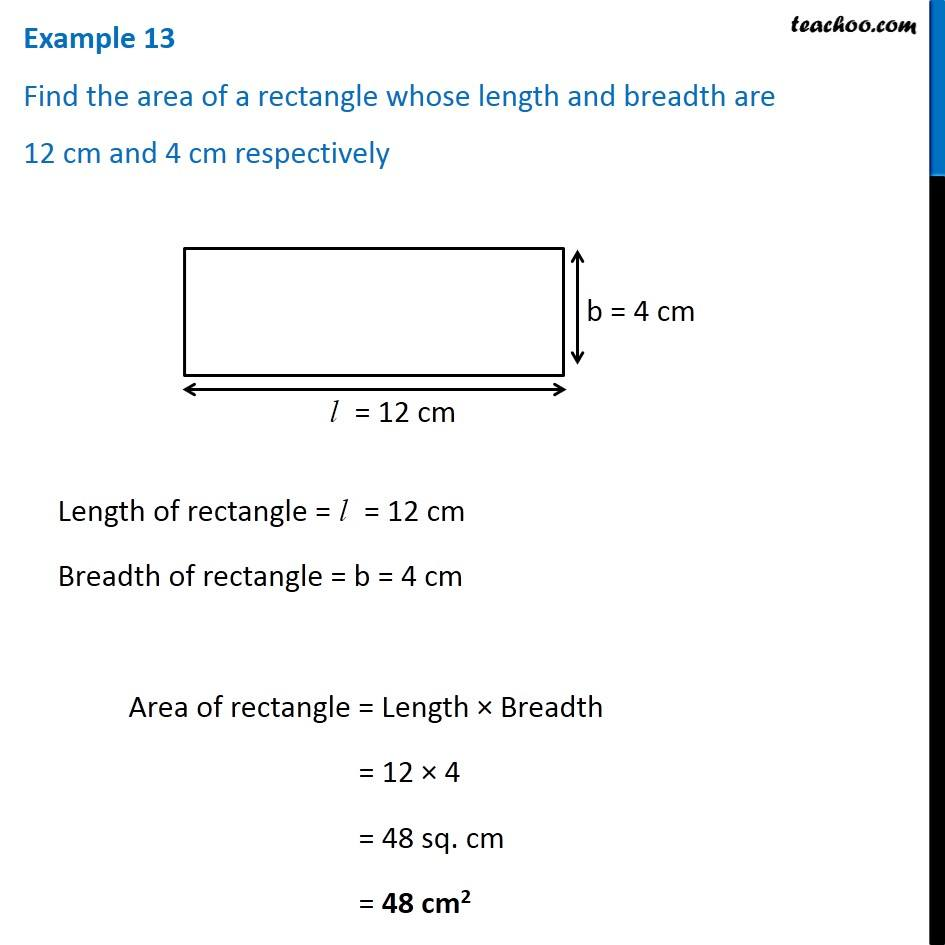1. Chapter 10 Class 6 Mensuration
2. Concept wise
3. Area of Rectangle

Transcript

Example 13 Find the area of a rectangle whose length and breadth are 12 cm and 4 cm respectively Length of rectangle = l = 12 cm Breadth of rectangle = b = 4 cm Area of rectangle = Length × Breadth = 12 × 4 = 48 sq. cm = 48 cm2